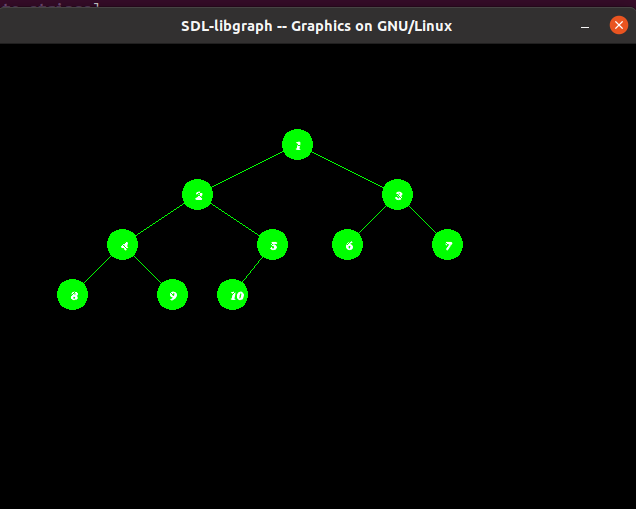Related Articles

# Represent Tree using graphics in C/C++

• Difficulty Level : Hard
• Last Updated : 05 May, 2021

Prerequisite: graphics.h, How to include graphics.h?

In C/C++ there is graphics.h header file which is used to create the object like line, circle, etc.
Given an array arr[] of N integers, the task is to write C++ program to create the Tree using graphics.h.

Approach: To run the program we have the include the below header file:

`#include `

We will create a Tree with the help below functions:

1. setcolor(color): This function present in graphic.h header file which is used to set the current drawing color to the new color.
2. floodfill(pattern, color): function is used to fill an enclosed area. The current fill pattern and fill color is used to fill the area.
3. circle(x, y, radius): The header file graphics.h contains circle() function which draws a circle with center at (x, y) and given radius.
4. outtextxy(): The header file graphics.h contains outtextxy() function which displays the text or string at a specified point (x, y) on the screen.

Below is the implementation of to draw Tree using graphics in C++:

## C++

 `// C++ program to draw the tree` `// in graphics.h` `#include ` `#include ` `#include ` `#include ` `using` `namespace` `std;`   `// Function that prints Tree using` `// functions graphic.h header file` `void` `printTree(``int` `x, ``int` `y, ``int``* array,` `               ``int` `index,` `               ``int` `total_elements)` `{`   `    ``// Base Case` `    ``if` `(index >= total_elements)` `        ``return` `NULL;`   `    ``// Convert int value into string` `    ``ostringstream str1;` `    ``str1 << array[index];`   `    ``string str2 = str1.str();` `    ``char``* str = &str2[0u];`   `    ``// Set color of the boundary of` `    ``// circle as green` `    ``setcolor(GREEN);`   `    ``// Draw the circle of radius 15` `    ``// that represent node of Tree` `    ``circle(x, y, 15);` `    ``floodfill(x, y, GREEN);`   `    ``// Print the values of the node` `    ``// in the circle` `    ``outtextxy(x - 2, y - 3, str);`   `    ``// Set the color of the line` `    ``// from parent to child as green` `    ``setcolor(GREEN);`   `    ``// Evaluating left and right child` `    ``int` `left = 2 * index + 1;` `    ``int` `right = 2 * index + 2;`   `    ``// Recursively draw the left subtree` `    ``// and the right subtree` `    ``printTree(x - y / (index + 1), y + 50,` `              ``array, left, total_elements);`   `    ``printTree(x + y / (index + 1), y + 50,` `              ``array, right, total_elements);`   `    ``// Draw the line (or link) when the` `    ``// node is not the leaf node` `    ``if` `(left < total_elements) {` `        ``line(x, y, x - y / (index + 1), y + 50);` `    ``}`   `    ``if` `(right < total_elements) {` `        ``line(x, y, x + y / (index + 1), y + 50);` `    ``}`   `    ``return` `NULL;` `}`   `// Driver Code` `int` `main()` `{` `    ``// Initialize graphic driver` `    ``int` `gd = DETECT, gm;` `    ``initgraph(&gd, &gm, ``"None"``);`   `    ``// Consider the tree as represented` `    ``/*` `             ``1` `          ``/     \` `         ``2       3` `       ``/  \     / \` `      ``4   5    6   7` `     ``/ \  /` `     ``8  9 10` `    ``*/`   `    ``// Given array arr[]` `    ``int` `array[] = { 1, 2, 3, 4, 5,` `                    ``6, 7, 8, 9, 10 };`   `    ``// Function Call` `    ``printTree(300, 100, array, 0, 10);` `    ``getch();`   `    ``// closegraph function closes the` `    ``// graphics mode and deallocates` `    ``// all memory allocated by` `    ``// graphics system` `    ``closegraph();` `}`

Output:Want to learn from the best curated videos and practice problems, check out the C++ Foundation Course for Basic to Advanced C++ and C++ STL Course for the language and STL. To complete your preparation from learning a language to DS Algo and many more, please refer Complete Interview Preparation Course.

My Personal Notes arrow_drop_up
Recommended Articles
Page :